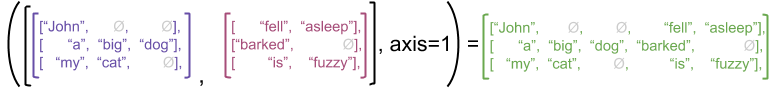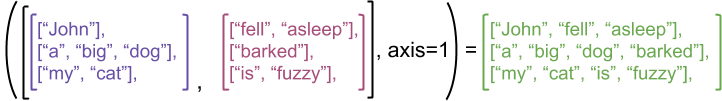# Text classification with RaggedTensors and Tensorflow Text

Prior to the introduction of TensorFlow Text, text pre-processing steps (cleaning, normalization, tokenization, encoding, etc.) were performed outside of TensorFlow runtime graph. This meant that potentially the pre-processing may differet between training and inference, for instance due to the use of different programming languages and runtimes to handle the task.

TensorFlow Text is a library introduced to provide native support for text for TensorFlow 2.0. With, TensorFlow Text text processing will be a step/operation in the TensorFlow graph and guaranteed to be the same during training and inference.

Also for efficiency, tokenized text sequences will be stored in RaggedTensor. Traditianlly text sequences of difference sizes were padded (e.g. with <pad> token) to form a fixed size tensor. This is required for any regular tensor operation like addition or multiplication. The resulting tensor would look like the following sourceWith a RaggedTensor padding is no longer required, this is due to the efficient way this kind of tensors store data. The resulting tensor would look like the following sourceThe rest of this post will explore how to use TensorFlow Text and RaggedTensors for a text classification task.

## Data

The data used in this post is IMDB review dataset which can be loaded as follows

(train_ds, valid_ds), test_ds = tfds.load(
name="imdb_reviews",
split=(tfds.Split.TRAIN.subsplit([8, 2]), tfds.Split.TEST),
as_supervised=True)


We need to create token-index table which will be used later during the creation of the RaggedTensor

# Create a lookup table for a vocabulary
vocab_values = tf.range(tf.size(vocab, out_type=tf.int64), dtype=tf.int64)
init = tf.lookup.KeyValueTensorInitializer(keys=vocab, values=vocab_values, key_dtype=tf.string, value_dtype=tf.int64)
vocab_table = tf.lookup.StaticVocabularyTable(init, num_oov, lookup_key_dtype=tf.string)


During the text pre-processing, we will use TensoFlow Text UnicodeScriptTokenizer to split text on whitespaces and also tokening punctuation. After that the lookup table will be used to encode tokens as follows:

def basic_preprocess(reviews, labels):
"""Perform basic preprocessing on the reviews text"""
# Lower case and normalize text
reviews = tftext.case_fold_utf8(reviews)
reviews = tftext.normalize_utf8(reviews, "NFD")
# Tokenize and encode the text
tokenizer = tftext.UnicodeScriptTokenizer()
rt = tokenizer.tokenize(reviews)
# Encode tokens
features = tf.ragged.map_flat_values(vocab_table.lookup, rt)

return features, labels


Then we apply the prepcessing on each dataset

train_ds = train_ds.batch(bs).map(basic_preprocess)
valid_ds = valid_ds.batch(bs).map(basic_preprocess)
test_ds = test_ds.batch(bs).map(basic_preprocess)


## Model

RaggedTensor cannot be used as is with any TensoFlow layer, in fact as of this writting they are supported by a handful of layers (e.g. Embedding):

First, an InputLayer flagged with ragged is used as the model input. This layer is followed by TensoFlow Text ToDense layer to pad-in inputs. After this, a regular Embedding layer is used to embed the tokens followed by an LSTM.

model = tf.keras.Sequential([
InputLayer(input_shape=(None,), dtype='int64', ragged=True),